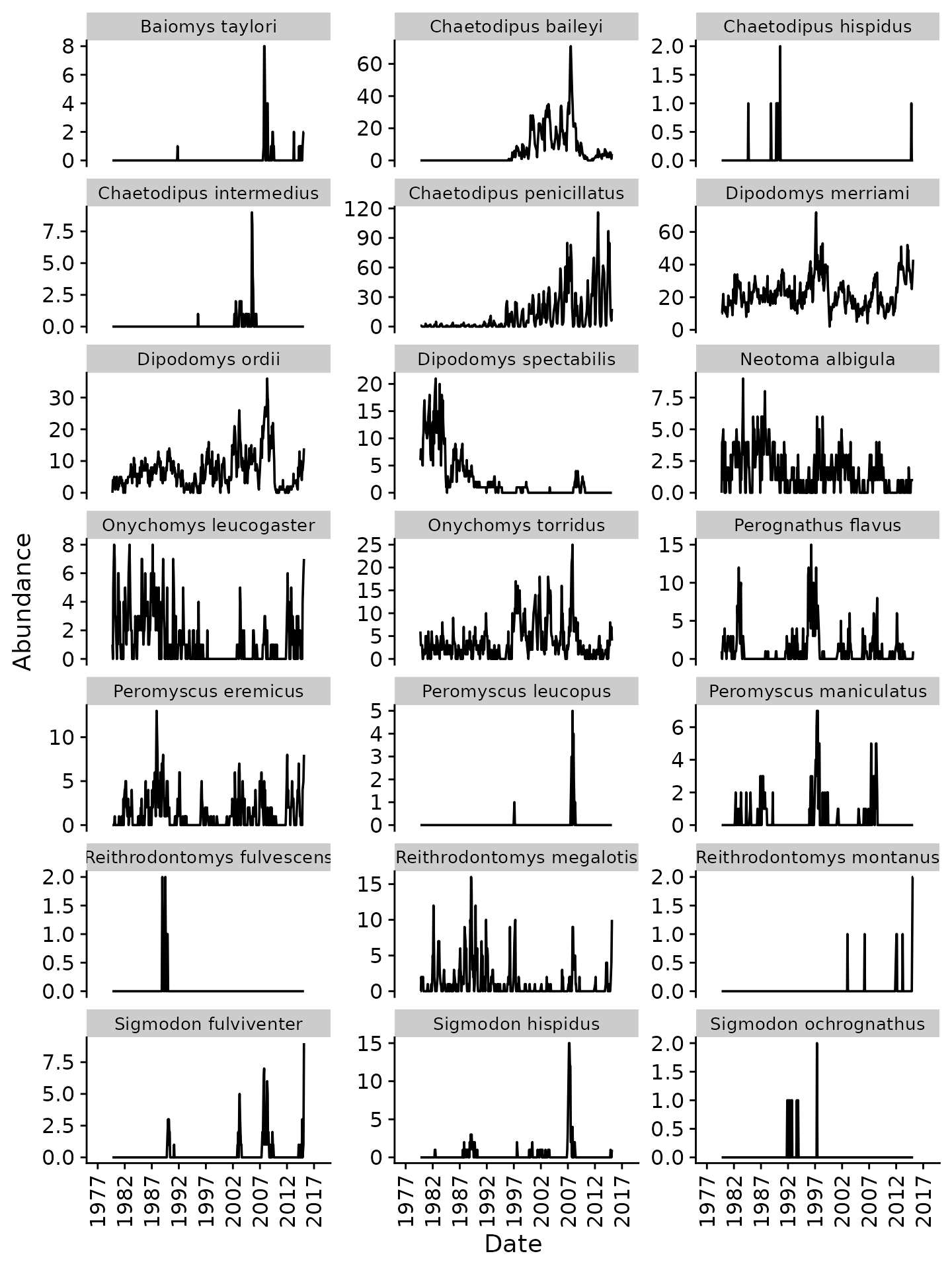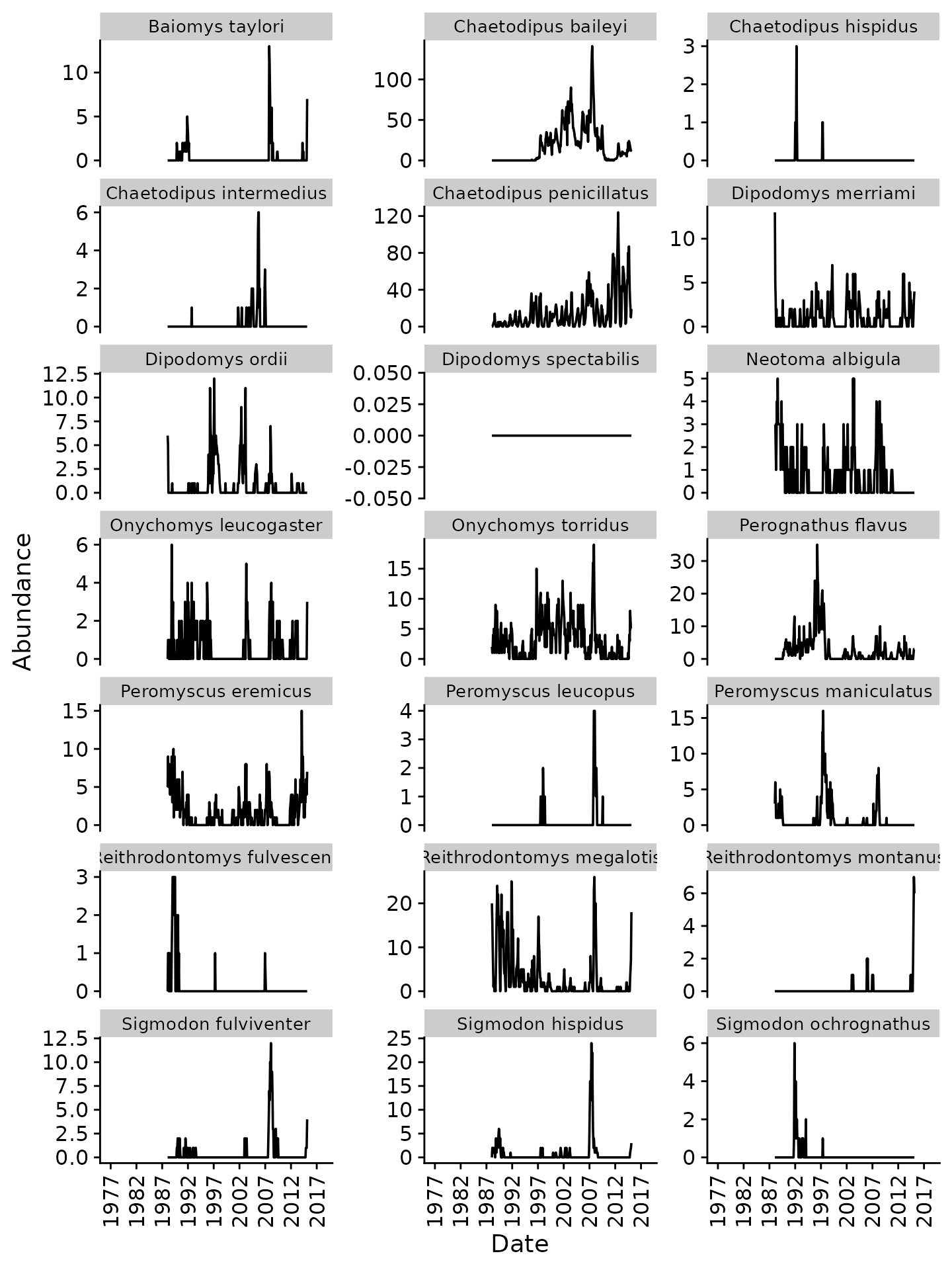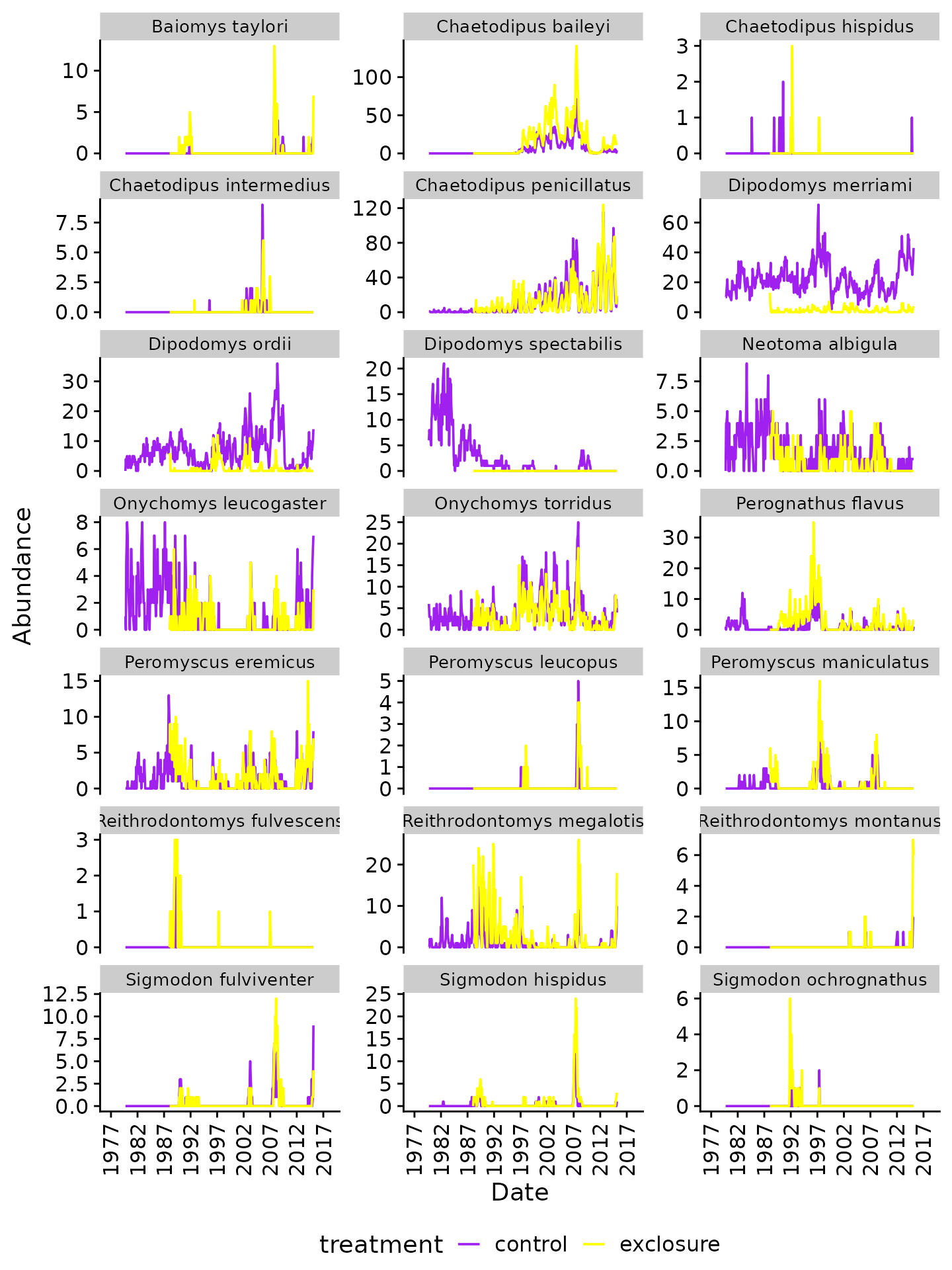Introduction

This vignette is a basic guide to begin exploring the Portal data. We load in the data (making sure that we’re using the most recent copy from GitHub), and then explore the rodent abundances over time, with a comparison between the “control” and “kangaroo rat exclosure” treatments.

Package Setup

• tidyverse contains several packages for data manipulation and plotting.

• cowplot contains a nicer default theme for ggplot.

• portalr is this package, which contains functions to read in the Portal data and do some basic processing.

library(tidyverse)
library(cowplot)
library(portalr)

Retrieving the Data

Note that this package does not contain the actual Portal data, which resides online in a GitHub repository.

First, we try to load the data. If there isn’t a Portal folder, than load_rodent_data will fall back to downloading the data, as well.

check whether we already have the data. If we don’t have the data, or if the version we have isn’t the most recent, we use the download_observations function to download the latest copy of the data.

portal_data_path <- tempdir() # use a temporary folder to store downloaded data
#> Loading in data version 1.115.0

The load_rodent_data function reads in several tables related to the rodent abundances. We won’t necessarily use all of these tables, but loading this now gives us access later.

print(summary(data_tables))
#>                Length Class      Mode
#> rodent_data    29     data.frame list
#> species_table  15     data.frame list
#> trapping_table  8     data.frame list
#> newmoons_table  4     data.frame list
#> plots_table     6     data.frame list

Rodent Abundances

The first table that we loaded (data_tables$rodent_data) is a record of whatever was found in the traps, mostly rodents, but also a few other taxa. If we just wanted to get the rodent abundance data, we could use the abundance function, which has default arguments to filter out the non-rodents. # get rodent abundance by plot rodent_abundance_by_plot <- abundance(path = portal_data_path, time = "date", level = "plot") #> Loading in data version 1.115.0 rodent_abundance <- rodent_abundance_by_plot %>% gather(species, abundance, -censusdate, -treatment, -plot) %>% count(species, censusdate, wt = abundance) %>% rename(abundance = n) print(summary(rodent_abundance)) #> species censusdate abundance #> Length:8715 Min. :1979-09-22 Min. : 0.000 #> Class :character 1st Qu.:1989-02-04 1st Qu.: 0.000 #> Mode :character Median :1997-09-27 Median : 0.000 #> Mean :1998-07-01 Mean : 6.755 #> 3rd Qu.:2008-04-06 3rd Qu.: 5.500 #> Max. :2018-05-19 Max. :285.000 Let’s convert the data to long format for easier facetting. Also, we want the scientific names instead of the two-letter species codes, so let’s do that matching, too: join_scientific_name <- function(rodent_abundance, species_table = data_tables$species_table)
{
return(rodent_abundance %>%
left_join(select(species_table, "species", "scientificname"),
by = "species") %>%
rename(scientific_name = scientificname)
)
}

rodent_abundance <- join_scientific_name(rodent_abundance)

Figure: abundance over time

make_abundance_plot_over_time <- function(rodent_abundance)
{
return(ggplot(rodent_abundance,
aes(x = censusdate, y = abundance)) +
geom_line() +
facet_wrap(~scientific_name, scales = "free_y", ncol = 3) +
xlab("Date") +
ylab("Abundance") +
scale_x_date(breaks = seq(as.Date("1977-01-01"), to = as.Date("2018-01-01"), "+5 years"),
date_labels = "%Y",
limits = as.Date(c("1977-01-01", "2018-01-01"))) +
theme_cowplot() +
theme(axis.text.x = element_text(angle = 90, hjust = 1, vjust = 0.5),
legend.position = "bottom", legend.justification = "center",
strip.text.x = element_text(size = 10))
)
}

my_plot <- make_abundance_plot_over_time(rodent_abundance)

print(my_plot)
#> Warning: Removed 3 rows containing missing values (geom_path).Monthly abundance of rodents (all plots)

Next Steps

Our next steps would likely be to dig deeper into the rodent abundances for different treatments, but first we want to know what the different treatments look like, so let’s revisit the abundances later.

Plot Treatments

A description of the experimental design and treatments can be found in this Readme file in the PortalDate repo.

For now, we are just going to use the Portal_plots table file to look at how the treatments for individual plots have changed over time. Note that this file is already loaded in as the plots_table from the loadData function we ran previously.

print(summary(data_tables$plots_table)) #> year month plot treatment #> Min. :1977 Min. : 1.000 Min. : 1.00 Length:10592 #> 1st Qu.:1988 1st Qu.: 4.000 1st Qu.: 6.00 Class :character #> Median :1997 Median : 7.000 Median :12.00 Mode :character #> Mean :1997 Mean : 6.513 Mean :12.48 #> 3rd Qu.:2008 3rd Qu.: 9.000 3rd Qu.:18.00 #> Max. :2018 Max. :12.000 Max. :24.00 #> resourcetreatment anttreatment #> Length:10592 Length:10592 #> Class :character Class :character #> Mode :character Mode :character #> #> #>  We want a proper date variable as well as converting plot into a factor: plot_treatments <- data_tables$plots_table %>%
mutate(iso_date = as.Date(paste0(year, "-", month, "-", "01")),
plot = as.factor(plot)) %>%
select(iso_date, plot, treatment)

Figure: plot treatments over time

my_plot <- ggplot(plot_treatments,
aes(x = iso_date, y = treatment, color = treatment)) +
geom_point(shape = 20) +
geom_vline(aes(xintercept = as.Date("1977-10-01")), linetype = 2) +
geom_vline(aes(xintercept = as.Date("1988-01-01")), linetype = 2) +
geom_vline(aes(xintercept = as.Date("2005-01-01")), linetype = 2) +
geom_vline(aes(xintercept = as.Date("2015-04-01")), linetype = 2) +
facet_wrap(~plot, ncol = 4) +
xlab("Date") +
ylab("Treatment") +
scale_color_manual(values = rainbow(4)) +
scale_x_date(breaks = seq(as.Date("1977-01-01"), to = as.Date("2018-01-01"), "+5 years"), date_labels = "%Y") +
theme_cowplot() +
guides(color = FALSE) +
theme(axis.text.x = element_text(angle = 90, hjust = 1, vjust = 0.5))

print(my_plot)Treatments of Plots by Date

Identifying control plots

The treatments for the plots have changed over time: in some cases, this was due to initial ramping up of the experimental protocol, in others, exclusions of Dipodomys spectabilis were started and then converted back later because the species went locally extinct (e.g. plots 1, 5, 9, 24).

Which plots have always been control plots?

always_control_plots <- plot_treatments %>%
group_by(plot) %>%
summarize(always_control = all(treatment == "control")) %>%
filter(always_control)

print(always_control_plots)
#> # A tibble: 4 x 2
#>   plot  always_control
#>   <fct> <lgl>
#> 1 4     TRUE
#> 2 11    TRUE
#> 3 14    TRUE
#> 4 17    TRUE

Note, however, that this excludes several plots for which the treatment was changed ~2015. We can include these plots by first filtering by date before testing for the “control” treatment:

mostly_control_plots <- plot_treatments %>%
filter(iso_date < "2015-01-01") %>%
group_by(plot) %>%
summarize(mostly_control = all(treatment == "control")) %>%
filter(mostly_control)

print(mostly_control_plots)
#> # A tibble: 8 x 2
#>   plot  mostly_control
#>   <fct> <lgl>
#> 1 2     TRUE
#> 2 4     TRUE
#> 3 8     TRUE
#> 4 11    TRUE
#> 5 12    TRUE
#> 6 14    TRUE
#> 7 17    TRUE
#> 8 22    TRUE

And to identify the datespan over which these plots have been controls:

date_span <- plot_treatments %>%
filter(plot %in% mostly_control_plots$plot) %>% group_by(iso_date) %>% summarize(all_control = all(treatment == "control")) %>% filter(all_control) print(date_span) #> # A tibble: 411 x 2 #> iso_date all_control #> <date> <lgl> #> 1 1977-07-01 TRUE #> 2 1977-08-01 TRUE #> 3 1977-09-01 TRUE #> 4 1977-10-01 TRUE #> 5 1977-11-01 TRUE #> 6 1977-12-01 TRUE #> 7 1978-01-01 TRUE #> 8 1978-02-01 TRUE #> 9 1978-03-01 TRUE #> 10 1978-04-01 TRUE #> # … with 401 more rows Abundances over control plots We are now ready to plot abundances just over the control plots and the time span in 1977-07-01 to 2015-03-01. We do this by retrieving the abundance data by plot, and then filtering accordingly: rodent_abundance_by_plot %>% filter(censusdate >= min(date_span$iso_date),
censusdate <= max(date_span$iso_date), plot %in% mostly_control_plots$plot) %>%
select(-treatment, -plot) %>%
gather(species, abundance, -censusdate) %>%
count(censusdate, species, wt = abundance) %>%
rename(abundance = n) %>%
join_scientific_name() %>%
{.} -> rodent_abundance_control

rodent_abundance_control %>%
make_abundance_plot_over_time() %>%
print()Monthly abundance of rodents (control plots)

Abundances over rodent exclosures

We can do the same with the “exclosure” condition. First, which plots:

mostly_exclosure_plots <- plot_treatments %>%
filter(iso_date > as.Date("1989-01-01"),
iso_date < "2015-01-01") %>%
group_by(plot) %>%
summarize(mostly_exclosure = all(treatment == "exclosure")) %>%
filter(mostly_exclosure)

print(mostly_exclosure_plots)
#> # A tibble: 8 x 2
#>   plot  mostly_exclosure
#>   <fct> <lgl>
#> 1 3     TRUE
#> 2 6     TRUE
#> 3 13    TRUE
#> 4 15    TRUE
#> 5 18    TRUE
#> 6 19    TRUE
#> 7 20    TRUE
#> 8 21    TRUE

Then, the datespan:

date_span <- plot_treatments %>%
filter(plot %in% mostly_exclosure_plots$plot) %>% group_by(iso_date) %>% summarize(all_exclosure = all(treatment == "exclosure")) %>% filter(all_exclosure) print(date_span) #> # A tibble: 297 x 2 #> iso_date all_exclosure #> <date> <lgl> #> 1 1988-01-01 TRUE #> 2 1988-02-01 TRUE #> 3 1988-03-01 TRUE #> 4 1988-04-01 TRUE #> 5 1988-05-01 TRUE #> 6 1988-06-01 TRUE #> 7 1988-07-01 TRUE #> 8 1988-08-01 TRUE #> 9 1988-09-01 TRUE #> 10 1988-10-01 TRUE #> # … with 287 more rows Finally, the figure: rodent_abundance_by_plot %>% filter(censusdate >= min(date_span$iso_date),
censusdate <= max(date_span$iso_date), plot %in% mostly_exclosure_plots$plot) %>%
select(-treatment, -plot) %>%
gather(species, abundance, -censusdate) %>%
count(censusdate, species, wt = abundance) %>%
rename(abundance = n) %>%
join_scientific_name() %>%
{.} -> rodent_abundance_exclosure

rodent_abundance_exclosure %>%
make_abundance_plot_over_time() %>%
print()Monthly abundance of rodents (exclosure plots)

Since these data have the same number of plots as the previous figure, we can directly compare abundances. Note the decreased numbers for kangaroo rats (Dipodomys spp.) and increased numbers for some other taxa.

Comparison Figure

Let’s merge the two datasets and produce a combined plot:

rodent_abundance_merged <- bind_rows(
mutate(rodent_abundance_control, treatment = "control"),
mutate(rodent_abundance_exclosure, treatment = "exclosure"))

merged_plot <- ggplot(rodent_abundance_merged,
aes(x = censusdate, y = abundance, color = treatment)) +
geom_line() +
facet_wrap(~scientific_name, scales = "free_y", ncol = 3) +
xlab("Date") +
ylab("Abundance") +
scale_x_date(breaks = seq(as.Date("1977-01-01"), to = as.Date("2018-01-01"), "+5 years"),
date_labels = "%Y",
limits = as.Date(c("1977-01-01", "2018-01-01"))) +
scale_color_manual(values = c("purple", "yellow")) +
theme_cowplot() +
theme(axis.text.x = element_text(angle = 90, hjust = 1, vjust = 0.5),
legend.position = "bottom", legend.justification = "center",
strip.text.x = element_text(size = 10))

print(merged_plot)Monthly abundance of rodents (control vs. exclosure plots)

As expected, there are substantially lower counts of kangaroo rats (Dipodomys spp.) in the “exclosure” plots. We also observe very similar abundances for some species, but increases in others (e.g. “Chaetodipus baileyi”, “Perognathus flavus”, “Reithrodontomys megalotis”)